﻿ 稳态对流扩散方程边值问题的一种有限元求解方法 A Finite Element Method for Solving the Boundary Value Problem of the Steady Convection-Diffusion Equation

Vol.05 No.01(2016), Article ID:17034,12 pages
10.12677/AAM.2016.51018

A Finite Element Method for Solving the Boundary Value Problem of the Steady Convection-Diffusion Equation

Jun Qiu, Shiju Yao, Hanquan Wang

School of Statistics and Mathematics, Yunnan University of Finance and Economics, Kunming YunnanReceived: Feb. 3rd, 2016; accepted: Feb. 22nd, 2016; published: Feb. 29th, 2016ABSTRACT

In this article, we aim to design a finite element method for solving the boundary value problem of the steady convection-diffusion equation. This boundary value problem is different from the general one, in which there is a small term in the equation, which will make us difficult to design a higher-order numerical method for such problem. We first design two standard finite element methods (including linear and quadratic finite element method) to solve this boundary value problem; we next use these two methods to obtain the approximated solution, and compare the approximated solution with the analytical one in Matlab; we finally propose suggestions to improve these two standard finite element methods based on nonuniform grids, in order to find a better approximation to the boundary value problem of the convection-diffusion equation.

Keywords:Steady Convection-Diffusion Equation, Boundary Value Problem, Finite Element Method, Nonuniform Grids, Boundary Layer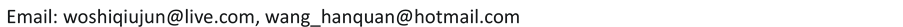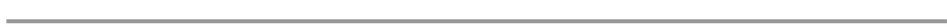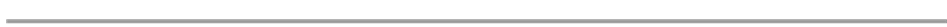1. 引言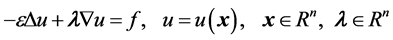(1)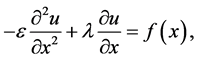(2)(3)

2. 有限元方法概述

2.1. 有限元法简介

2.2. 有限元法设计的基本思路

2.3. 有限元方法的解题基本步骤 

1) 通过变分原理将微分方程转化为变分形式的方程

2) 单元剖分：将待解区域进行分割，离散成有限个子区间的集合，每一个子区间就称为一个单元，单元的形状原则上可以是任意的，二维问题一般采用三角形单元或矩形单元，三维空间可采用四面体或多面体等，每个单元的顶点称为节点。

3) 有限元离散：在单元剖分的基础上，然后分割单元中任意点的未知函数用该分割单元中形状函数及离散网格点上的函数值展开，即建立一个插值函数，最后利用分段插值函数构造有限维子空间，通过它来离散变分形式的方程，构造有限元方程。

4) 求解近似变分方程：在前两部分的基础上最后进行约束处理，形成有限元法的代数方程组，再从该方程组求解函数在各节点上的近似值。

3. 对流扩散问题变分化

1)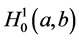表示所有定义在区间[a,b]上具有一阶连续导数的函数；

2)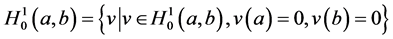(4)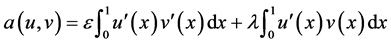(5)(6)

1) 梯形法法(求积)准则：(7)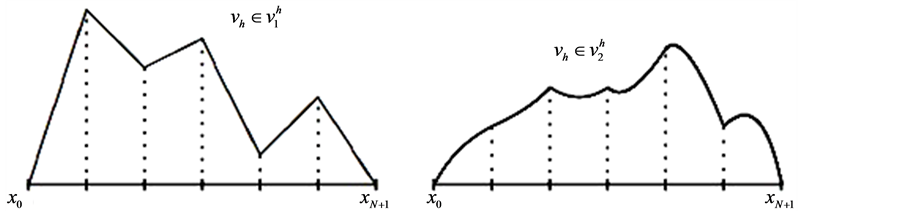(a) (b)

Figure 1. Examples of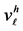: (a)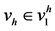and (b)2) 辛普森正交法(求积)准则：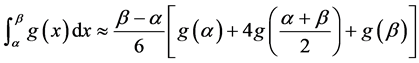(8)

4. 两种有限元方法的求解过程

1) 基于一次元的有限元法，简记为P1，它在每个单元上用一次多项式来近似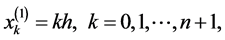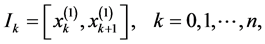(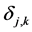是Kronecker符号)，需要注意的是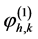是作用于相连的两个区间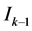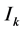。在有限元方法中，点被称为节点，区间被称为单元格。(9)(10)Figure 2. Function Space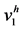hat Function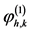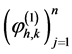的基础上我们也可以展开函数，得到近似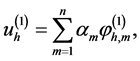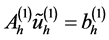(11)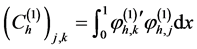2) 基于一次元的有限元法，简记为P2，它在每个单元上用二次多项式来近似当整数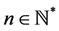，我们先定义下列节点：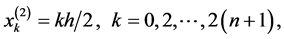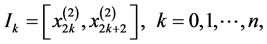(12)

(12)是我们建立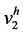的基础。对于每一个单元格区间,基于节点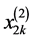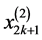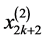这三个相连点，我们定义如下三个二次拉格朗日多项式来联系：(13)(14)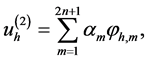(15)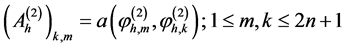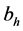是向量空间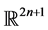上的一个向量，它的基本元定义为：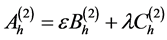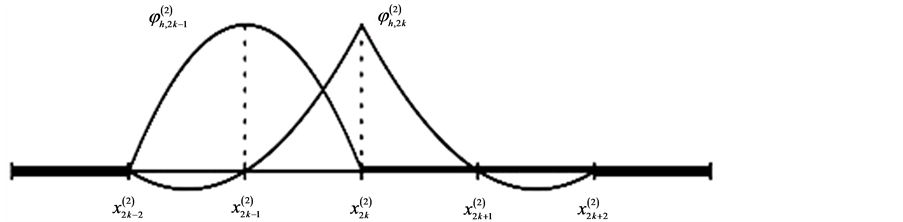Figure 3. A hat function on Function Spaces5. 数值计算结果1) 当n = 10的情况下运行Matlab程序，其画图结果如图4

2) 当n = 20的情况下运行Matlab程序，其画图结果如图5

1) 当n = 10的情况下运行Matlab程序，其画图结果如图6

2) 当n = 20的情况下运行Matlab程序，其画图结果如图7

1) 当n = 10的情况下运行Matlab程序，其画图结果如图8

2) 当n = 20的情况下运行Matlab程序，其画图结果如图9

1) 当n = 10的情况下运行Matlab程序，其画图结果如图10

2) 当n = 20的情况下运行Matlab程序，其画图结果如图11

6. 方法的改进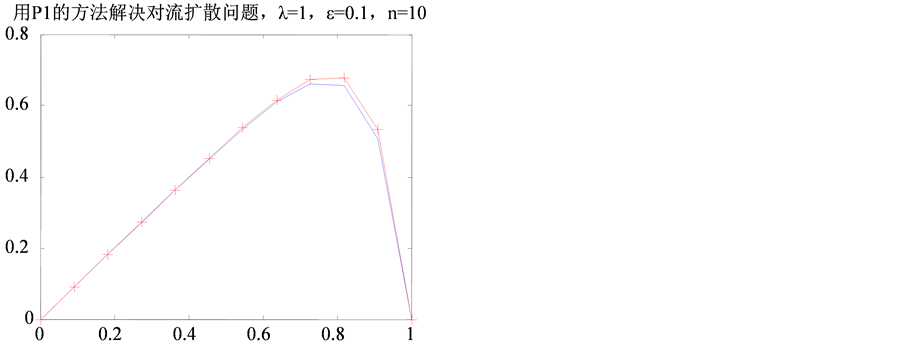Figure 4. Approximate solution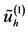(red) and exact solution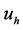(blue)Figure 5. Approximate solution(red) and exact solution(blue)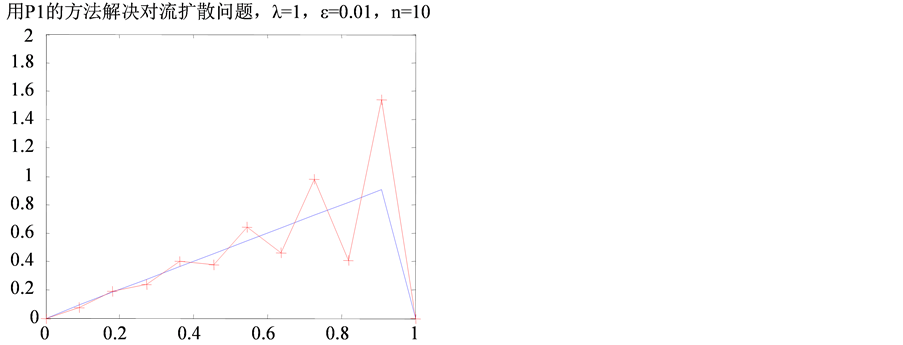Figure 6. Approximate solution(red) and exact solution(blue)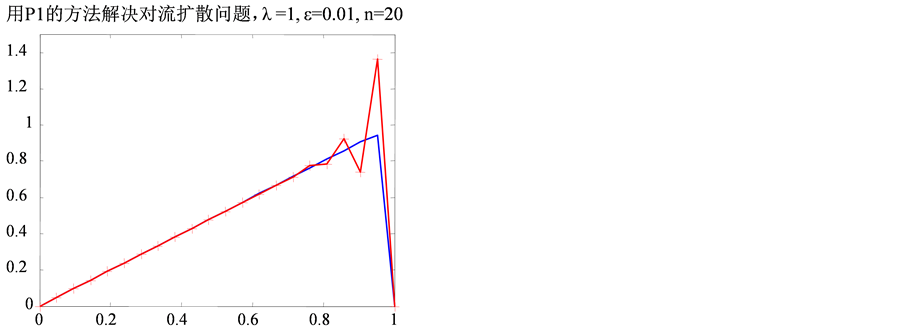Figure 7. Approximate solution(red) and exact solution(blue)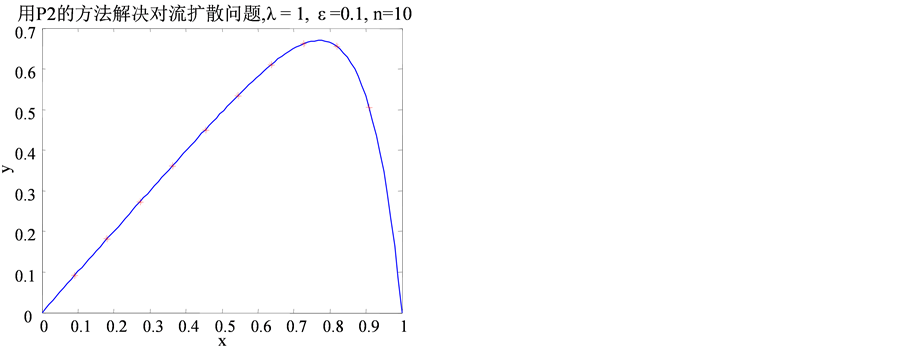Figure 8. Approximate solution(red) and exact solution(blue)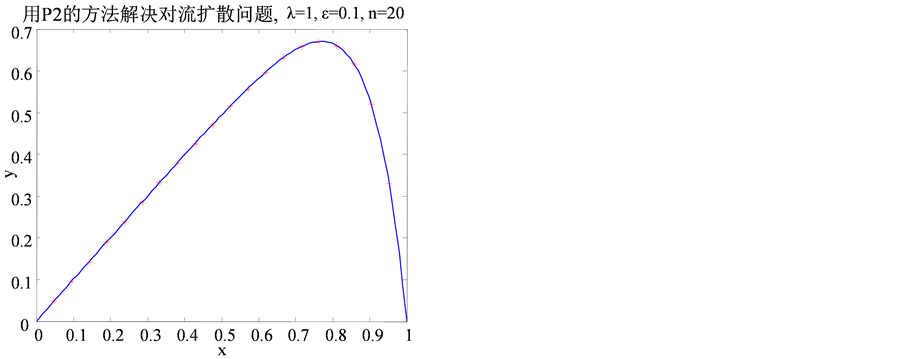Figure 9. Approximate solution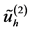(red) and exact solution(blue)Figure 10. Approximate solution(red) and exact solution(blue)Figure 11. Approximate solution(red) and exact solution(blue)

7. 总结

A Finite Element Method for Solving the Boundary Value Problem of the Steady Convection-Diffusion Equation[J]. 应用数学进展, 2016, 05(01): 131-142. http://dx.doi.org/10.12677/AAM.2016.51018

1. 1. Atkinson, K. and Han, W. (2001) Theoretical Numerical Analysis. Springer, New York.

2. 2. 陆金甫, 关治. 偏微分数值解法[M]. 第二版. 北京: 清华大学出版社, 2008.

3. 3. Bernardi, C., Maday, Y. and Rapetti, F. (2004) Discréti-sations variationnelles de problèmes aux limites elliptiques. Collection S.M.A.I. Mathematiques et, Applications. Springer, Paris, Vol. 45.

4. 4. 李人宪. 有限元法基础[M]. 第二版. 北京: 国防工业出版社, 2004.

5. 5. 毕超. 计算流体力学有限元方法及其编程详解[M]. 北京: 机械工业出版社, 2013.

6. 6. Brezzi, F. and Russo, A. (1994) Choosing Bubbles for Advection-Diffusion Problems. Mathematical Models and Methods in Applied Sciences, 4, 571. http://dx.doi.org/10.1142/S0218202594000327

7. 7. 王焕定, 陈少峰, 边文凤. 有限单元法基础及MATLAB编程[M]. 北京: 高等教育出版社, 2012.

8. 8. Lucquin, B. and Pironneau, O. (1998) Introduction to Scientific Computing. Willey, Chichester.